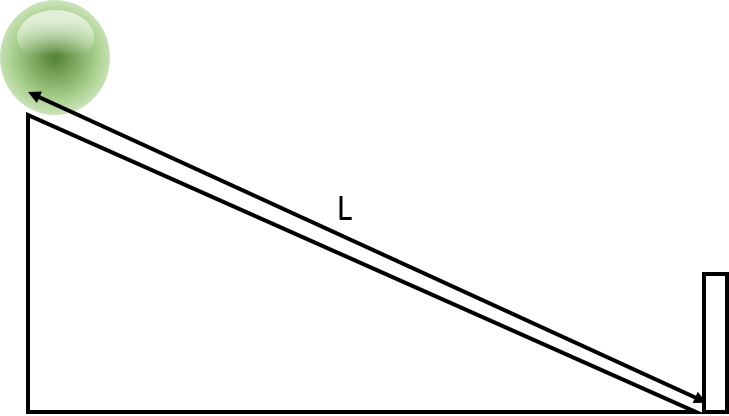# The story of a ball and a slope SJPO questionAs shown in the figure above, a ball slides along a friction-less ramp of length $L$. As it reaches the bottom, it hits the board and slides up along the slope again, which it continues to do until it comes to rest.

If the speed of the ball after the collision is $\frac { 4 }{ 5 }$ times the speed of the ball just before the collision, find the total distance traveled by the ball when it comes to a complete stop.

The total distance traveled by the ball can be expressed as $\frac { a }{ b } L$. What is $a+b$?

Extra fact: This is one of the easiest problems from the 2012 SJPO Special Round with a rating of only 5m.

This question is part of my set SJPO Practice Questions.

×

Problem Loading...

Note Loading...

Set Loading...